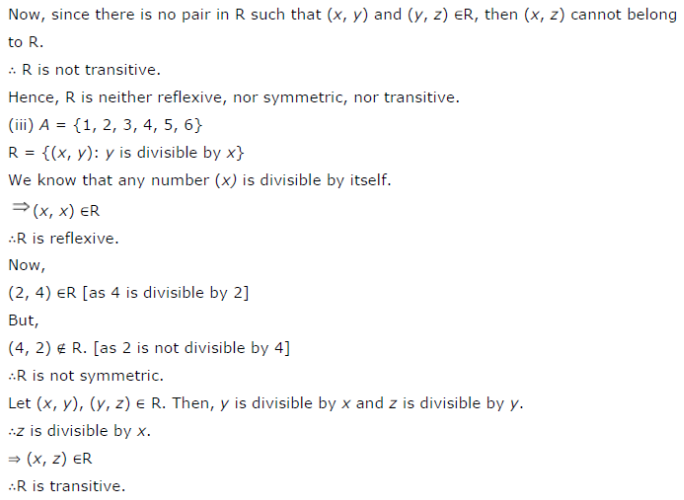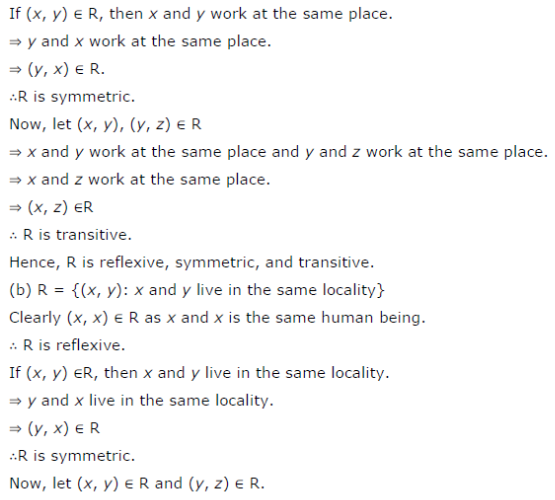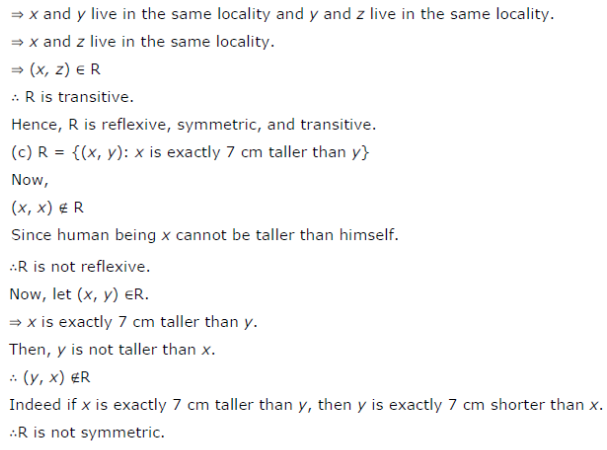# Determine whether each of the following relations are reflexive, symmetric and transitive

Determine whether each of the following relations are reflexive, symmetric and transitive:
(i) Relation R in the set A = {1, 2, 3,13, 14} defined as
R = {(x, y): 3x − y = 0}
(ii) Relation R in the set N of natural numbers defined as
R = {(x, y): y = x + 5 and x < 4}
(iii) Relation R in the set A = {1, 2, 3, 4, 5, 6} as
R = {(x, y): y is divisible by x}
(iv) Relation R in the set Z of all integers defined as
R = {(x, y): x − y is as integer}
(v) Relation R in the set A of human beings in a town at a particular time given by
(a) R = {(x, y): x and y work at the same place}
(b) R = {(x, y): x and y live in the same locality}
(c ) R = {(x, y): x is exactly 7 cm taller than y}
(d) R = {(x, y): x is wife of y}
(e) R = {(x, y): x is father of y}